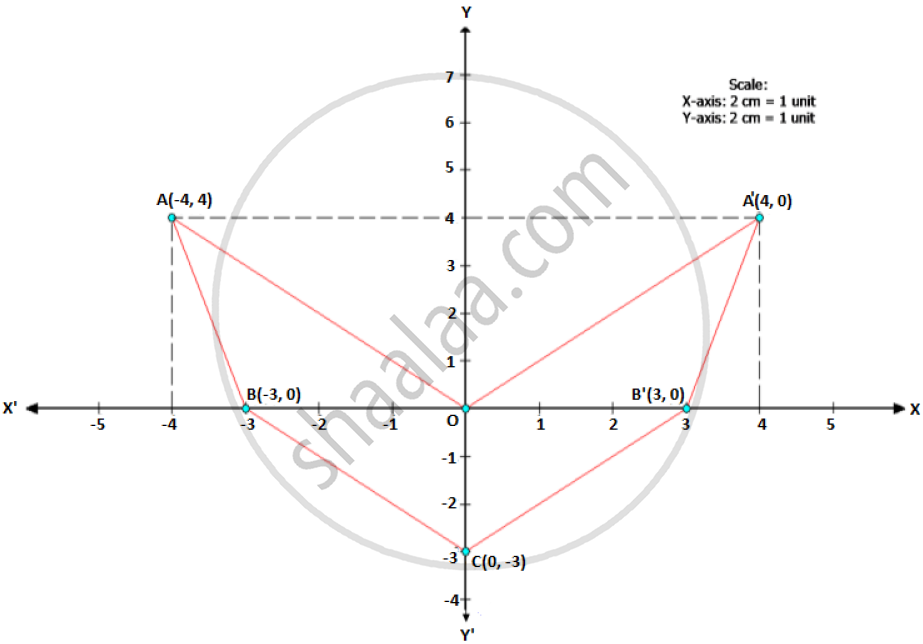Share

# Use Graph Paper for this Question. (Take 2 Cm = 1 Unit Along Both X and Y-axis.) Plot the Points O(0, 0), A(-4, 4), B(-3, 0) and C(0, -3) and Reflect Points a and B on the Y-axis and Name Them A’ and B’ Respectively. Write Down Their Coordinates. and Name the Figure Oabcb’A’. - ICSE Class 10 - Mathematics

#### Question

Use graph paper for this question. (Take 2 cm = 1 unit along both x and y-axis.) Plot the points O(0, 0), A(-4, 4), B(-3, 0) and C(0, -3)

1) Reflect points A and B on the y-axis and name them A’ and B’ respectively. Write down their coordinates.

2) Name the figure OABCB’A’.

3) State the line of symmetry of this figure

#### Solution

1)A’ = (4, 4) AND B’ = (3, 0)

2) The figure is an arrowhead

3) The y-axis is the line of symmetry of figure OABCB’A’.

Is there an error in this question or solution?

#### APPEARS IN

2015-2016 (March) (with solutions)
Question 4.2 | 4.00 marks

#### Video TutorialsVIEW ALL 

Solution Use Graph Paper for this Question. (Take 2 Cm = 1 Unit Along Both X and Y-axis.) Plot the Points O(0, 0), A(-4, 4), B(-3, 0) and C(0, -3) and Reflect Points a and B on the Y-axis and Name Them A’ and B’ Respectively. Write Down Their Coordinates. and Name the Figure Oabcb’A’. Concept: Lines of Symmetry.
S